Documentation

# cluster

## Syntax

``T = cluster(Z,'Cutoff',C)``
``T = cluster(Z,'Cutoff',C,'Depth',D)``
``T = cluster(Z,'Cutoff',C,'Criterion',criterion)``
``T = cluster(Z,'MaxClust',N)``

## Description

````T = cluster(Z,'Cutoff',C)` defines clusters from an agglomerative hierarchical cluster tree `Z`. The input `Z` is the output of the `linkage` function for an input data matrix `X`. `cluster` cuts `Z` into clusters, using `C` as a threshold for the inconsistency coefficients (or `inconsistent` values) of nodes in the tree. The output `T` contains cluster assignments of each observation (row of `X`).```

example

````T = cluster(Z,'Cutoff',C,'Depth',D)` evaluates `inconsistent` values by looking to a depth `D` below each node.```

example

````T = cluster(Z,'Cutoff',C,'Criterion',criterion)` uses either `'inconsistent'` (default) or `'distance'` as the `criterion` for defining clusters. `criterion` must be less than `C` for `cluster` to define clusters.```

example

````T = cluster(Z,'MaxClust',N)` defines a maximum of `N` clusters using `'distance'` as the criterion for defining clusters.```

## Examples

collapse all

Perform agglomerative clustering on randomly generated data by evaluating inconsistent values to a depth of four below each node.

Randomly generate the sample data.

```rng('default'); % For reproducibility X = [(randn(20,2)*0.75)+1; (randn(20,2)*0.25)-1];```

Create a scatter plot of the data.

```scatter(X(:,1),X(:,2)); title('Randomly Generated Data');```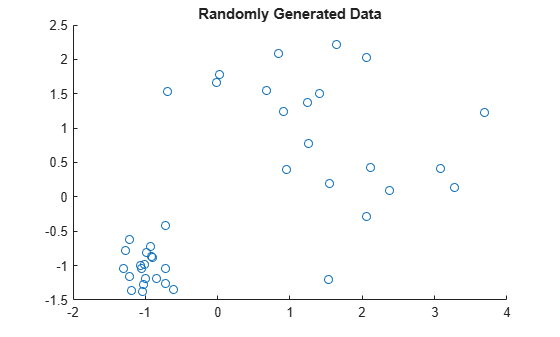Create a hierarchical cluster tree using the `ward` linkage method.

`Z = linkage(X,'ward');`

Create a dendrogram plot of the data.

`dendrogram(Z)`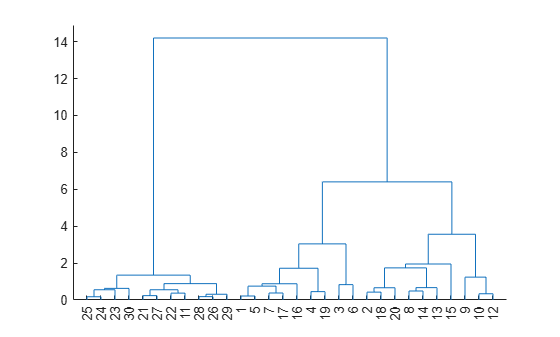The scatter plot and the dendrogram plot seem to show two clusters in the data.

Cluster the data using a threshold of 3 for the inconsistency coefficient and looking to a depth of 4 below each node. Plot the resulting clusters.

```T = cluster(Z,'cutoff',3,'Depth',4); gscatter(X(:,1),X(:,2),T)```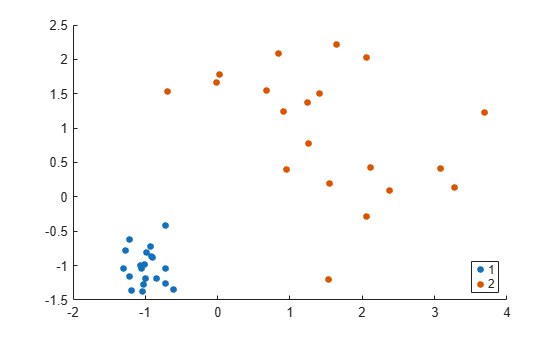`cluster` identifies two clusters in the data.

Perform agglomerative clustering on the `fisheriris` data set using `'distance'` as the criterion for defining clusters. Visualize the cluster assignments of the data.

Load the `fisheriris` data set.

`load fisheriris`

Visualize a 2-D scatter plot of the data using species as the grouping variable. Specify marker colors and marker symbols for the three different species.

```gscatter(meas(:,1),meas(:,2),species,'rgb','do*') title("Actual Clusters of Fisher's Iris Data")```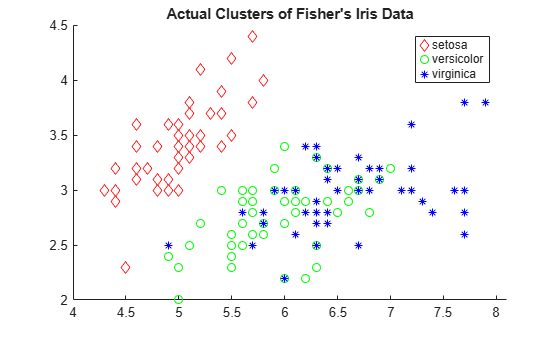Create a hierarchical cluster tree using the `'average'` method and the `'chebychev'` metric.

`Z = linkage(meas,'average','chebychev');`

Cluster the data using a threshold of 1.5 for the `'distance'` criterion.

`T = cluster(Z,'cutoff',1.5,'Criterion','distance')`
```T = 150×1 2 2 2 2 2 2 2 2 2 2 ⋮ ```

`T` contains numbers that correspond to the cluster assignments. Find the number of classes that `cluster` identifies.

`length(unique(T))`
```ans = 3 ```

`cluster` identifies three classes for the specified values of `cutoff` and `Criterion`.

Visualize a 2-D scatter plot of the clustering results using `T` as the grouping variable. Specify marker colors and marker symbols for the three different classes.

```gscatter(meas(:,1),meas(:,2),T,'rgb','do*') title("Cluster Assignments of Fisher's Iris Data")```Clustering correctly identifies the setosa class (class 2) as belonging to a distinct cluster, but poorly distinguishes between the versicolor and virginica classes (classes 1 and 3, respectively). Note that the scatter plot labels the classes using the numbers contained in `T`.

Find a maximum of three clusters in the `fisheriris` data set and compare cluster assignments of the flowers to their known classification.

`load fisheriris`

Create a hierarchical cluster tree using the `'average'` method and the `'chebychev'` metric.

`Z = linkage(meas,'average','chebychev');`

Find a maximum of three clusters in the data.

`T = cluster(Z,'maxclust',3);`

Create a dendrogram plot of `Z`. To see the three clusters, use `'ColorThreshold'` with a cutoff halfway between the third-from-last and second-from-last linkages.

```cutoff = median([Z(end-2,3) Z(end-1,3)]); dendrogram(Z,'ColorThreshold',cutoff)```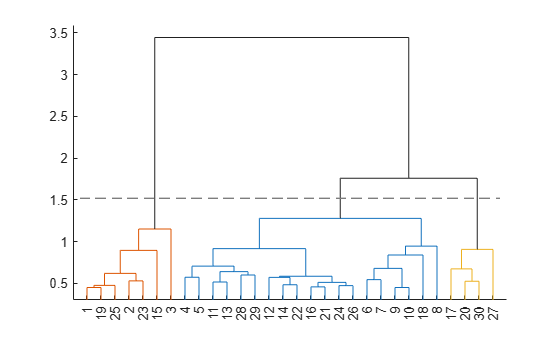Display the last two rows of `Z` to see how the three clusters are combined into one. `linkage` combines the 293rd (blue) cluster with the 297th (red) cluster to form the 298th cluster with a linkage of `1.7583`. `linkage` then combines the 296th (green) cluster with the 298th cluster.

`lastTwo = Z(end-1:end,:)`
```lastTwo = 2×3 293.0000 297.0000 1.7583 296.0000 298.0000 3.4445 ```

See how the cluster assignments correspond to the three species. For example, one of the clusters contains `50` flowers of the second species and `40` flowers of the third species.

`crosstab(T,species)`
```ans = 3×3 0 0 10 0 50 40 50 0 0 ```

Randomly generate sample data with 20,000 observations.

```rng('default') % For reproducibility X = rand(20000,3);```

Create a hierarchical cluster tree using the `ward` linkage method. In this case, the `'SaveMemory'` option of the `clusterdata` function is set to `'on'` by default. In general, specify the best value for `'SaveMemory'` based on the dimensions of `X` and the available memory.

`Z = linkage(X,'ward');`

Cluster the data into a maximum of four groups and plot the result.

```c = cluster(Z,'Maxclust',4); scatter3(X(:,1),X(:,2),X(:,3),10,c)```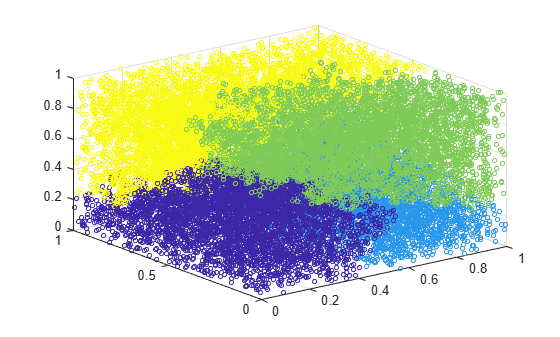`cluster` identifies four groups in the data.

## Input Arguments

collapse all

Agglomerative hierarchical cluster tree that is the output of the `linkage` function, specified as a numeric matrix. For an input data matrix `X` with m rows (or observations), `linkage` returns an (m – 1)-by-3 matrix `Z`. For an explanation of how `linkage` creates the cluster tree, see `Z`.

Example: `Z = linkage(X)`, where `X` is an input data matrix

Data Types: `single` | `double`

Threshold for defining clusters, specified as a positive scalar or a vector of positive scalars. `cluster` uses `C` as a threshold for either the heights or the inconsistency coefficients of nodes, depending on the `criterion` for defining clusters in a hierarchical cluster tree.

• If the criterion for defining clusters is `'distance'`, then `cluster` groups all leaves at or below a node into a cluster, provided that the height of the node is less than `C`.

• If the criterion for defining clusters is `'inconsistent'`, then the `inconsistent` values of a node and all its subnodes must be less than `C` for `cluster` to group them into a cluster. `cluster` begins from the root of the cluster tree `Z` and steps down through the tree until it encounters a node whose `inconsistent` value is less than the threshold `C`, and whose subnodes (or descendants) have inconsistent values less than `C`. Then `cluster` groups all leaves at or below the node into a cluster (or a singleton if the node itself is a leaf). `cluster` follows every branch in the tree until all leaf nodes are in clusters.

Example: `cluster(Z,'Cutoff',0.5)`

Data Types: `single` | `double`

Depth for computing inconsistent values, specified as a numeric scalar. `cluster` evaluates inconsistent values by looking to a depth `D` below each node.

Example: `cluster(Z,'Cutoff',0.5,'Depth',3)`

Data Types: `single` | `double`

Criterion for defining clusters, specified as `'inconsistent'` or `'distance'`.

If the criterion for defining clusters is `'distance'`, then `cluster` groups all leaves at or below a node into a cluster (or a singleton if the node itself is a leaf), provided that the height of the node is less than `C`. The height of a node in a tree represents the distance between the two subnodes that are merged at that node. Specifying `'distance'` results in clusters that correspond to a horizontal slice of the `dendrogram` plot of `Z`.

If the criterion for defining clusters is `'inconsistent'`, then `cluster` groups a node and all its subnodes into a cluster, provided that the inconsistency coefficients (or `inconsistent` values) of the node and subnodes are less than `C`. Specifying `'inconsistent'` is equivalent to `cluster(Z,'Cutoff',C)`.

Example: `cluster(Z,'Cutoff',0.5,'Criterion','distance')`

Data Types: `char` | `string`

Maximum number of clusters to form, specified as a positive integer or a vector of positive integers. `cluster` constructs a maximum of `N` clusters, using `'distance'` as the criterion for defining clusters. The height of each node in the tree represents the distance between the two subnodes merged at that node. `cluster` finds the smallest height at which a horizontal cut through the tree will leave `N` or fewer clusters. See Specify Arbitrary Clusters for more details.

Example: `cluster(Z,'MaxClust',5)`

Data Types: `single` | `double`

## Output Arguments

collapse all

Cluster assignment, returned as a numeric vector or matrix. For the (m – 1)-by-3 hierarchical cluster tree `Z` (the output of `linkage` given input `X`), `T` contains the cluster assignments of the m rows (observations) of `X`.

The size of `T` depends on the corresponding size of `C` or `N`.

• If `C` is a positive scalar, then `T` is a vector of length m.

• If `N` is a positive integer, then `T` is a vector of length m.

• If `C` is a length l vector of positive scalars, then `T` is an m-by-l matrix with one column per value in `C`.

• If `N` is a length l vector of positive integers, then `T` is an m-by-l matrix with one column per value in `N`.

## Alternative Functionality

If you have an input data matrix `X`, you can use `clusterdata` to perform agglomerative clustering and return cluster indices for each observation (row) in `X`. The `clusterdata` function performs all the necessary steps for you, so you do not need to execute the `pdist`, `linkage`, and `cluster` functions separately.